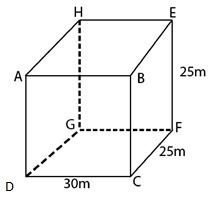# A small indoor greenhouse (herbarium) is made entirely of glass panes (including base) held together with tape. It is 30cm long, 25 cm wide and 25 cm high. (i) What is the area of the glass? (ii) How much of tape is needed for all the 12 edges?

Given

Length of greenhouse (l) = 30 cm

Breadth of greenhouse (b) = 25 cm

Height of greenhouse (h) = 25 cm

Find out

We have to find the

(i)What is the area of the glass?

(ii)How much tape is needed for all the 12 edges?

Solution

(i) Area of glass = total surface area of greenhouse

= 2 (lb + bh + lh)

= 2 (30 * 25 + 25 * 25 + 30 * 25)

= 2 (750 + 625 + 750)

= 4250 cm2

Therefore, area of glass is 4250 cm2.

(ii) Length of tape needed = perimeter of the greenhouse= 4 (l + b + h)

= 4 (30 + 25 + 25) cm

= 320 cm.

Therefore, the length of tape needed is 320 cm.(51)(7)